# Test: Linear Equations- 2

## 15 Questions MCQ Test General Test Preparation for CUET | Test: Linear Equations- 2

Description
Attempt Test: Linear Equations- 2 | 15 questions in 15 minutes | Mock test for GMAT preparation | Free important questions MCQ to study General Test Preparation for CUET for GMAT Exam | Download free PDF with solutions
QUESTION: 1

### For what value of N would the following equation have no solution? 3(4x−7)+12=2(5x−3)+N(x−3)

Solution:

Simplify both sides of the equation as much as possible, and solve for x in the equation in terms of N:

3(4x−7)+12=2(5x−3)+N(x−3)
3⋅4x−3⋅7+12=2⋅5x−2⋅3+N⋅x−N⋅3
12x−21+12=10x−6+Nx−3N
12x−9=(10+N)x+(−6−3N)
12x−(10+N)x=(−6−3N)+9
(2−N)x=3−3N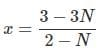x has exactly one solution unless the denominator is 0 - that is, N=2. We make sure that this value renders no solution by substituting:

3(4x−7)+12=2(5x−3)+N(x−3)
3(4x−7)+12=2(5x−3)+2(x−3)
12x−21+12=10x−6+2x−6
12x−9=12x−12
−9=−12

The equation has no solution, and N=2 is the correct answer.

QUESTION: 2

Solution:

Explanation:
n+2=−14−n
n+n=−14−2
2n=−16
n=−8

QUESTION: 3

### Solve for x: −6x−20=−2x+4(1−3x)

Solution:

Explanation:
−6x−20=−2x+4(1−3x
−6x−20=−2x+4−12x
−6x−20=−14x+4
−6x+14x=4+20
8x=24
x=3

QUESTION: 4

Solve for b: −14+6b+7−2b=1+5b

Solution:

−14+6b+7−2b=1+5b
−7+4b=1+5b
4b−5b=1+7
−b=8
b=−8

QUESTION: 5

What is the midpoint coordinate of (1,4) and (7,10)?

Solution:

Midpoint formula: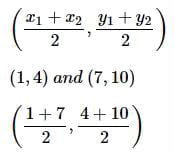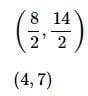QUESTION: 6

What is the midpoint coordinate of (1,2) and (5,2)?

Solution:

Midpoint formula: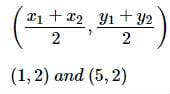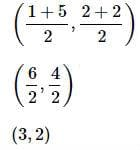QUESTION: 7

What is the midpoint coordinate of (−2,−1) and (−8,7)?

Solution:

Midpoint formula: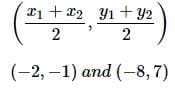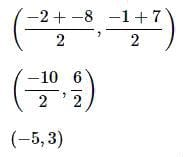QUESTION: 8

Solve the following equation:

2|x−5|+16=30.

Solution:

We start by isolating the absolute value expression:

2|x−5|+16=30⇔2|x−5|=30−16=14⇔|x−5|=7

This gives us two cases when we remove the absolute value:

x−5=7 and x−5=−7

Then we solve for each case:

x−5=7⇒x=7+5⇒x=12

x−5=−7⇒x=−7+5⇒x=−2

QUESTION: 9

Solve for N:

5(N−6)−2(N+4)=7(N+5)

Solution:

5(N−6)−2(N+4)=7(N+5)
(5N−30)−(2N+8)=7N+35
5N−30−2N−8=7N+35
3N−38=7N+35
3N−38+38=7N+35+38
3N=7N+73
3N−7N=7N+73−7N
−4N=73
N= 73/-4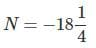QUESTION: 10

Solve for x:

(4x+7)+2(x+15)=3(x−17)

Solution:

(4x+7)+2(x+15)=3(x−17)
(4x+7)+(2x+30)=3x−51
6x+37=3x−51
6x+37−3x=3x−51−3x
3x+37=−51
3x=−51−37
3x=−88
x= -88/3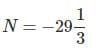QUESTION: 11

Which of the following equations has the set of all real numbers as its solution set?

Solution:

The right side of each equation is 8(N+3), which simplifies by way of distribution to

8(N+3)=8⋅N+8⋅3=8N+24

If the left side of the equation simplifies to an identical expression, the equation has all real numbers as its solutions.

We test the left side of each equation:

2(N+4)+6N=8(N+3)
2(N+4)+6N=2⋅N+2⋅4+6N=2N+8+6N=8N+8

3(N+4)+5N=8(N+3)

3(N+4)+5N=3⋅N+3⋅4+5N=3N+12+5N=8N+12

4(N+4)+4N=8(N+3)

4(N+4)+4N=4⋅N+4⋅4+4N=4N+16+4N=8N+16

5(N+4)+3N=8(N+3)

5(N+4)+3N=5⋅N+5⋅4+3N=5N+20+3N=8N+20

6(N+4)+2N=8(N+3)

6(N+4)+2N=6⋅N+6⋅4+2N=6N+24+2N=8N+24

Of the given choices,

6(N+4)+2N=8(N+3)

can be rewritten as

8N+24=8N+24,

which is an identity and has the set of all real numbers as its solution set.

QUESTION: 12

Consider the incomplete equation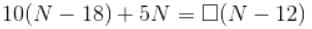What number replaces the box in order to form an equation with no solution?

Solution:

Set A to be the number that replaces the box.
Simplify first:
10(N−18)+5N=A(N−12)
10N−180+5N=A(N−12)
15N−180=AN−12A
15N−180+180−AN = AN−12A+180−AN
15N−AN=180−12A
Now solve for N in terms of A:
(15−A)N=180−12A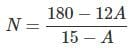The only possible value of A that might preclude the existence of a solution is A=15, since it makes the denominator 0. However, let us test this value in the original equation:
10(N−18)+5N=15(N−12)
15N−180=15N−180
As it turns out, replacing the box with 15 yields an identity, not a contradiction, so the solution set is the set of all real numbers. There is no number that fits the description.

QUESTION: 13

Consider the incomplete equation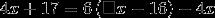Which of the following numbers can replace the box to form an equation whose one and only solution is 2?

Solution:

Rewrite this equation as

4x+17=6(Ax−16)−4x

If 2 is a solution of the equation, then we can substitute 2 for x to make a true arithmetic equation. Replace x with 2 and solve for A:

4⋅2+17=6(A⋅2−16)−4⋅2

8+17=6(2A−16)−8

25 = 12 A - 96 - 8
25=12A−104

129=12A
A=129/12
This number replaces the box in order to form the equation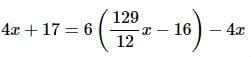QUESTION: 14

Solve the following equation for x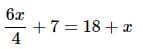Solution:

We proceed as follows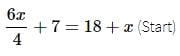6x+28=72+4x  (Multiply both sides by 4. Remember to distribute the 4 to both summands on both sides.)
6x=44+4x (Subtract 28 from both sides)
2x=44 (Subtract 4x from both sides)
x=22 (Divide both sides by 2)

QUESTION: 15

Define a function f as follows:

f(x)=8x−35

If f(N)=47, evaluate N.

Solution:

Since f(N)=47, we can plug N in for x and 47 in for f(N) to get the following equation,
8N−35=47
From here, we want to solve for N therefore we must isolate N on one side of the equation and all other numbers on the other side.
8N=47+35
8N=82
N=82÷8
N=10.25Use Code STAYHOME200 and get INR 200 additional OFF Use Coupon Code